# Understanding Quadrilaterals- Introduction to Geometry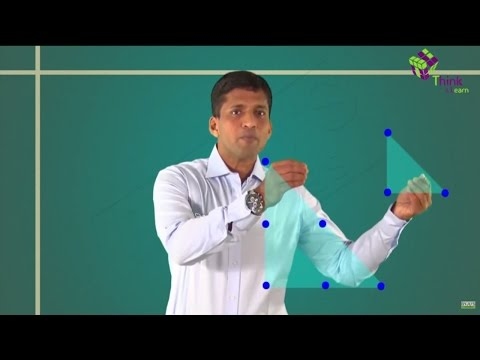Geometry is derived from the ancient Greek terms geo and metron, which means earth and measurement respectively. It is a branch of mathematics dealing with shape, size, position, spatial relationships and properties of figures.

A point is a primitive notion upon which geometry is built according to Euclidean geometry. A point can be visualized as a circle with zero radii. A point has zero dimensions and can specify position or location. It has no length and is represented by a dot.

If a point is moved in a random manner as shown, then it forms a curved line. But if the point is moved in such a way that it does not change its direction then a straight line is obtained.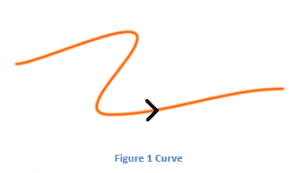A line is a one-dimensional collection of points, which extends infinitely in both the directions. A line has no thickness and it is straight.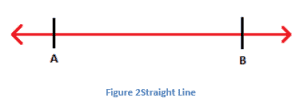$$\begin{array}{l}A\end{array}$$
line can uniquely be specified using two points. Suppose, a line passing through points
$$\begin{array}{l}A\end{array}$$
and
$$\begin{array}{l}B\end{array}$$
is denoted as
$$\begin{array}{l}\overleftrightarrow{AB}\end{array}$$
. The arrows indicate that line
$$\begin{array}{l}\overleftrightarrow{AB}\end{array}$$
extends infinitely.

The line segment is any portion of a line, which has two end points. Also, a portion of any line with only one endpoint is called a ray. A line segment with

$$\begin{array}{l}A\end{array}$$
and
$$\begin{array}{l}B\end{array}$$
as two endpoints is represented as
$$\begin{array}{l}\overline{AB}\end{array}$$
. The figure 2 shown above represents a line segment
$$\begin{array}{l}\overline{AB}\end{array}$$
and the two arrows at the end indicate a line.

If we rotate ray about its end-point, the measure of its rotation is called angle between its initial and final position.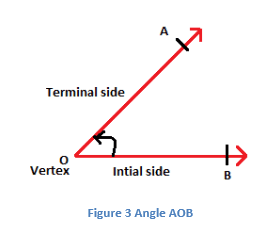A polygon is defined as a plane figure, which is bounded by finite line segments to form a closed figure. A polygon with 5 sides is known as a pentagon, 6 sided polygon is the hexagon, 7 sided polygon is known as heptagon and so on. Based on the number of sides or vertices a polygon can be defined as n-sided polygon.The sum of interior angles of any n-sided polygon is given by

$$\begin{array}{l}(n-2)~×~180°\end{array}$$
.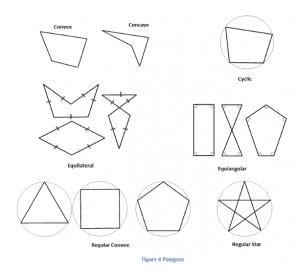As polygons are closed figures and the minimum number of sides required for a plane figure to be closed is 3, the polygon with the least number of sides is the triangle.It has three edges and three vertices.

Fig. 5 represents a triangle with three sides

$$\begin{array}{l}AB\end{array}$$
,
$$\begin{array}{l}BC\end{array}$$
,
$$\begin{array}{l}CA\end{array}$$
and three vertices
$$\begin{array}{l}A\end{array}$$
,
$$\begin{array}{l}B\end{array}$$
and
$$\begin{array}{l}C\end{array}$$
. The sum of interior angles of a triangle is
$$\begin{array}{l}180°\end{array}$$
.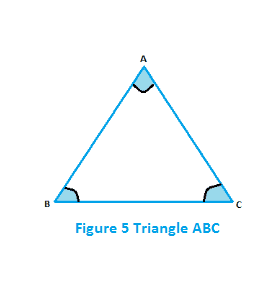A 4-sided polygon is known as a quadrilateral. The sum of interior angles of a quadrilateral is

$$\begin{array}{l}360°\end{array}$$
.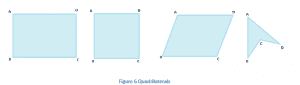A diagonal is a line segment connecting the 2 non-consecutive vertices of a polygon.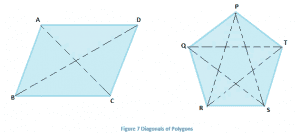In fig. 7,

$$\begin{array}{l}ABCD\end{array}$$
is a quadrilateral and in this case
$$\begin{array}{l}A\end{array}$$
and
$$\begin{array}{l}C\end{array}$$
are 2 non-consecutive vertices, so
$$\begin{array}{l}AC\end{array}$$
represents a diagonal. Similarly,
$$\begin{array}{l}B\end{array}$$
and
$$\begin{array}{l}D\end{array}$$
are non-consecutive so
$$\begin{array}{l}BD\end{array}$$
also represents a diagonal. Similarly, in pentagon
$$\begin{array}{l}PQRST\end{array}$$
, if all the non-consecutive vertices are joined then diagonals will be obtained. In this case,
$$\begin{array}{l}PR\end{array}$$
,
$$\begin{array}{l}PS\end{array}$$
,
$$\begin{array}{l}TR\end{array}$$
,
$$\begin{array}{l}TQ\end{array}$$
and
$$\begin{array}{l}QS\end{array}$$
represent the diagonals.

Based on diagonals, polygons can be classified as convex and concave.

In the case of convex polygons, diagonals always lie inside the boundary of the polygon.   In the concave polygons, one or more diagonals or a portion of it lies outside the boundary of a polygon and also one or more interior angle of a polygon is the reflex.

In fig. 8, the first figure represents a convex polygon, (ii) is concave polygons as diagonal BD lies outside and

$$\begin{array}{l}∠C\end{array}$$
is the reflex angle. Similarly, in third polygon diagonal
$$\begin{array}{l}AD\end{array}$$
lies outside and
$$\begin{array}{l}∠E\end{array}$$
is the reflex, so it is a concave polygon.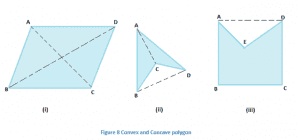Based on sides and angles polygons can be classified as regular or irregular polygons. The polygons, which have equal sides and equal interior angles are regular polygons and those having any unequal side or angle are known as irregular polygons.

For example, A square is a regular polygon as all its sides are of the same length and also the measure of each interior angle is

$$\begin{array}{l}90°\end{array}$$
,, an equilateral triangle is also a regular polygon. A rectangle, on the other hand, is irregular as the measure of its sides is not equal even though interior angles being same.

Let us consider a quadrilateral PQRS in the figure below: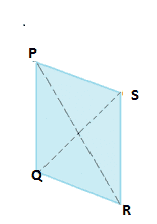• PQ, QR, RS and SP are the four sides.
• Points P, Q, R, and S are the four vertices.
• ∠PQR, ∠QRS, ∠RSP and ∠SPR are the four angles.
• PQ and QR are the opposite sides.
• ∠P and ∠R are the opposite angles.
• PQ and QR are the adjacent sides.
• ∠P and ∠Q are the adjacent angles.

Geometry thus is a field where one could barely miss the topic of quadrilaterals as most students would have studied them during their secondary school days. But to implement what they have learned,  students should practice more with the kind of sample questions given here- NCERT solutions for understanding quadrilaterals.